# PostgreSQL Tutorial: GROUP BY

August 1, 2023

Summary: in this tutorial, you will learn how to divide rows into groups by using the PostgreSQL `GROUP BY` clause.

## Introduction to PostgreSQL `GROUP BY` clause

The `GROUP BY` clause divides the rows returned from the `SELECT` statement into groups. For each group, you can apply an aggregate function e.g., `SUM()` to calculate the sum of items or `COUNT()` to get the number of items in the groups.

The following statement illustrates the basic syntax of the `GROUP BY` clause:

``````SELECT
column_1,
column_2,
...,
aggregate_function(column_3)
FROM
table_name
GROUP BY
column_1,
column_2,
...;
``````

In this syntax:

• First, select the columns that you want to group e.g., `column1` and `column2`, and column that you want to apply an aggregate function (`column3`).
• Second, list the columns that you want to group in the `GROUP BY` clause.

The statement clause divides the rows by the values of the columns specified in the `GROUP BY` clause and calculates a value for each group.

It’s possible to use other clauses of the `SELECT` statement with the `GROUP BY` clause.

PostgreSQL evaluates the `GROUP BY` clause after the `FROM` and `WHERE` clauses and before the `HAVING` `SELECT`, `DISTINCT`, `ORDER BY` and `LIMIT` clauses.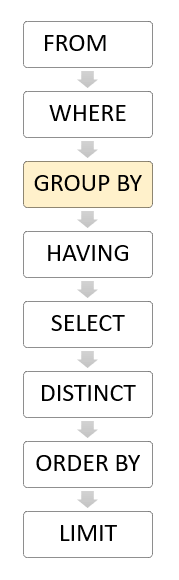## PostgreSQL `GROUP BY` clause examples

Let’s take a look at the `payment` table in the sample database.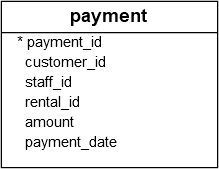### 1) Using PostgreSQL `GROUP BY` without an aggregate function example

You can use the `GROUP BY` clause without applying an aggregate function. The following query gets data from the `payment` table and groups the result by customer id.

``````SELECT
customer_id
FROM
payment
GROUP BY
customer_id;
``````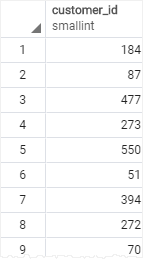In this case, the `GROUP BY` works like the `DISTINCT` clause that removes duplicate rows from the result set.

### 2) Using PostgreSQL `GROUP BY` with `SUM()` function example

The `GROUP BY` clause is useful when it is used in conjunction with an aggregate function.

For example, to select the total amount that each customer has been paid, you use the `GROUP BY` clause to divide the rows in the `payment` table into groups grouped by customer id. For each group, you calculate the total amounts using the `SUM()` function.

The following query uses the `GROUP BY` clause to get the total amount that each customer has been paid:

``````SELECT
customer_id,
SUM (amount)
FROM
payment
GROUP BY
customer_id;
``````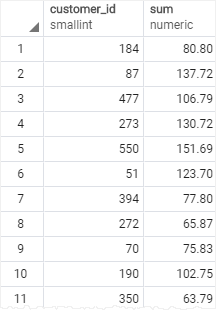The `GROUP BY` clause sorts the result set by customer id and adds up the amount that belongs to the same customer. Whenever the `customer_id` changes, it adds the row to the returned result set.

The following statement uses the `ORDER BY` clause with `GROUP BY` clause to sort the groups:

``````SELECT
customer_id,
SUM (amount)
FROM
payment
GROUP BY
customer_id
ORDER BY
SUM (amount) DESC;
``````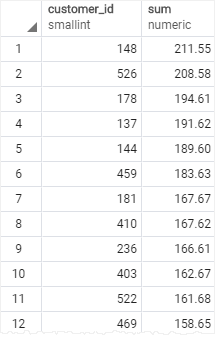### 3) Using PostgreSQL GROUP BY clause with the JOIN clause

The following statement uses the `GROUP BY` clause with the `INNER JOIN` clause the get the total amount paid by each customer.

Unlike the previous example, this query joins the `payment` table with the `customer` table and group customers by their names.

``````SELECT
first_name || ' ' || last_name full_name,
SUM (amount) amount
FROM
payment
INNER JOIN customer USING (customer_id)
GROUP BY
full_name
ORDER BY amount DESC;
``````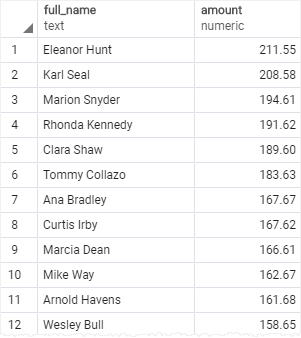### 4) Using PostgreSQL `GROUP BY` with `COUNT()` function example

To find the number of payment transactions that each staff has processed, you group the rows in the `payment` table by the values in the `staff_id` column and use the `COUNT()` function to get the number of transactions:

``````SELECT
staff_id,
COUNT (payment_id)
FROM
payment
GROUP BY
staff_id;
``````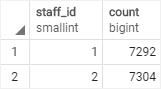The `GROUP BY` clause divides the rows in the payment into groups and groups them by value in the `staff_id` column. For each group, it returns the number of rows by using the `COUNT()` function.

### 5) Using PostgreSQL `GROUP BY` with multiple columns

The following example uses multiple columns in the `GROUP BY` clause:

``````SELECT
customer_id,
staff_id,
SUM(amount)
FROM
payment
GROUP BY
staff_id,
customer_id
ORDER BY
customer_id;
``````

In this example, the `GROUP BY` clause divides the rows in the `payment` table by the values in the `customer_id` and `staff_id` columns. For each group of `(customer_id, staff_id)`, the `SUM()` calculates the total amount.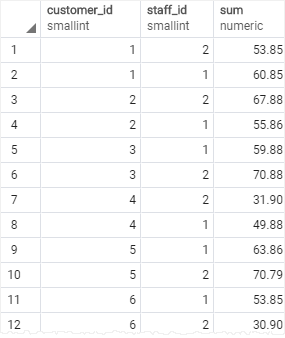### 6) Using PostgreSQL GROUP BY clause with a date column

The `payment_date` is a timestamp column. To group payments by dates, you use the `DATE()` function to convert timestamps to dates first and then group payments by the result date:

``````SELECT
DATE(payment_date) paid_date,
SUM(amount) sum
FROM
payment
GROUP BY
DATE(payment_date);
``````In this tutorial, you have learned how to use the PostgreSQL `GROUP BY` clause to divide rows into groups and apply an aggregate function to each group.